## UGC NET CS 2017 Jan- paper-3

 Question 1
Which of the following is an interrupt according to temporal relationship with system clock?
 A Maskable interrupt B Periodic interrupt C Division by zero D Synchronous interrupt
Computer-Organization       Interruption
Question 1 Explanation:
Synchronous interrupts are produced by the CPU control unit while executing instructions and are called synchronous because the control unit issues them only after terminating the execution of an instruction.
This interrupt according to temporal relationship with system clock.
Asynchronous interrupts are generated by other hardware devices at arbitrary times with respect to the CPU clock signals..
Maskable Interrupts - Those interrupts whose request can be denied by microprocessor.
eg- RST 1, RST2, RST 5, RST 6.5 etc..
Non maskable Interrupts - Interrupts whose request cannot be denied. eg -RST 4.5 or TRAP ,RESET IN.
. Periodic interrupts to cause a software task to be executed on a periodic basis.
 Question 2
Which of the following is incorrect for virtual memory?
 A Large programs can be written B More I/O is required C More addressable memory available D Faster and easy swapping of process
Operating-Systems       Virtual Memory
Question 2 Explanation:
Option-A TRUE: Implementing virtual memory is need to write large program
Option-B TRUE: It require more I/O
Option-C FALSE: Not more addressable memory available.
Option-D TRUE: Virtual memory is faster and easy swapping of process.
 Question 3
The general configuration of the micro-programmed control unit is given below:What are blocks B and C in the diagram respectively?
 A Block address register and cache memory B Control address register and control memory C Branch register and cache memory D Control address register and random access memory
Computer-Organization       Microprogrammed-Control-Unit
Question 3 Explanation:Question 4
Match the following :A a-(iv), b-(iii), c-(i), d-(ii) B a-(iv), b-(i), c-(iii), d-(ii) C a-(iv), b-(ii), c-(i), d-(iii) D a-(iv), b-(iii), c-(ii), d-(i)
Question 4 Explanation:
Implied → Specified implicitly in the definition of instruction
Immediate→ Registers which are in CPU
Register→ Specified in the register
Register Indirect→ Specified implicitly in the definition of instruction
 Question 5
In 8085 microprocessor, the digit 5 indicates that the microprocessor needs:
 A –5 volts, +5 volts supply B +5 volts supply only C –5 volts supply only D 5 MHz clock
Computer-Organization       Microprocessor
Question 5 Explanation:
Before 8085 microprocessors require +5V, -5V and 12V. In 8085 microprocessor need +5 volts supply only.
 Question 6
In 8085, which of the following performs : load register pair immediate operation?
 A LDAX rp B LHLD addr C LXI rp, data D INX rp
Computer-Organization       Microprocessor
Question 6 Explanation:
LDAX: Load accumulator indirect( This instruction copies the contents of that memory location into the accumulator. )
LHLD: Load H and L register direct ( This instruction loads the contents of the 16- bit memory location into the H and L register pair. )
LXI: Load register pair immediate( The instruction loads 16-bit data in the register pair designated in the operand.)
INX: Increment register pair by 1.( It will increment the register value by 1.)
 Question 7

Consider following schedules involving two transactions:

S1 : r1(X); r1(Y); r2(X); r2(Y); w2(Y); w1(X)

S2 : r1(X); r2(X); r2(Y); w2(Y); r1(Y); w1(X)

Which of the following statement is true?
 A Both S1 and S2 are conflict serializable. B S1 is conflict serializable and S2 is not conflict serializable. C S1 is not conflict serializable and S2 is conflict serializable. D Both S1 and S2 are not conflict serializable.
Database-Management-System       Transactions
Question 7 Explanation:Question 8
Which one is correct w.r.t. RDBMS ?
 A primary key ⊆ super key ⊆ candidate key B primary key ⊆ candidate key ⊆ super key C super key ⊆ candidate key ⊆ primary key D super key ⊆ primary key ⊆ candidate key
Database-Management-System       Relational-databases
Question 8 Explanation:Question 9
Let pk(R) denotes primary key of relation R. A many-to-one relationship that exists between two relations R1 and R2 can be expressed as follows :
 A pk(R2) → pk(R1) B pk(R1) → pk(R2) C pk(R2) → R1 ∩ R2 D pk(R1) → R1 ∩ R2
Database-Management-System       Keys
Question 9 Explanation:
Consider an example, where we have two entities Employee(whose primary key is R1) and Department(whose primary key is R2) and a department have many employees and each employee works in one department i.e. many to one relationship.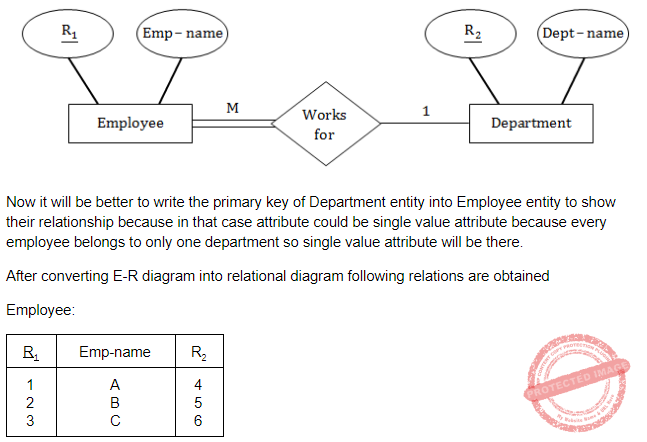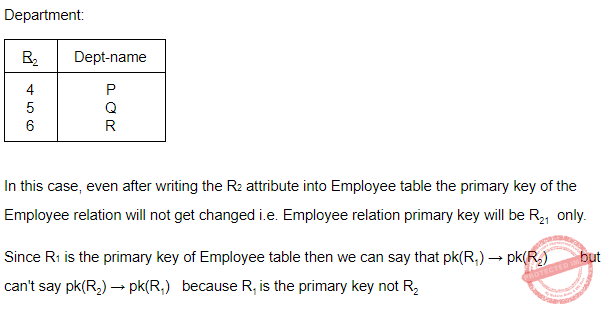Question 10
For a database relation R(A, B, C, D) where the domains of A, B, C and D include only atomic values, only the following functional dependencies and those that can be inferred from them are :
A → C
B → D
The relation R is in _______.
 A First normal form but not in second normal form. B Both in first normal form as well as in second normal form. C Second normal form but not in third normal form. D Both in second normal form as well as in third normal form.
Database-Management-System       Normalization
Question 10 Explanation:
Candidate key is ab.
Since all a, b, c, d are atomic. So the relation is in 1NF.
Checking the FD's
a → c
b → d
We can see that there is partial dependencies. So it is not 2NF.
 Question 11
Works (emp name, company name, salary)
Here, emp_name is primary key. Consider the following SQL query
Select emp name
From works T
Where salary > (select avg (salary)
from works S
where T.company _ name = S.company _name)
The above query is for following :
 A Find the highest paid employee who earns more than the average salary of all employees of his company B Find the highest paid employee who earns more than the average salary of all the employees of all the companies. C Find all employees who earn more than the average salary of all employees of all the companies. D Average salary of all employees of their company.
Database-Management-System       SQL
Question 11 Explanation: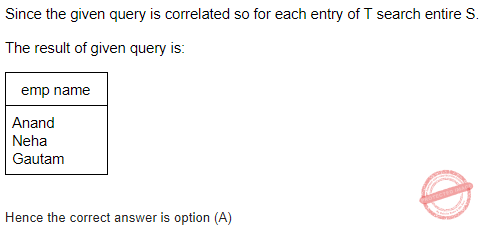Question 12
If following sequence of keys are inserted in a B+ tree with K(=3) pointers: 8, 5, 1, 7, 3, 12, 9, 6 Which of the following shall be correct B+ tree?
 ABCDDatabase-Management-System       B-and-B+-Trees
Question 12 Explanation: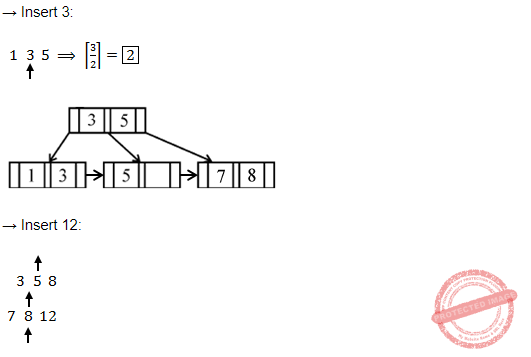Question 13
Which of the following statement(s) is/are correct ?
 A Persistence is the term used to describe the duration of phosphorescence. B The control electrode is used to turn the electron beam on and off. C The electron gun creates a source of electrons which are focused into a narrow beam directed at the face of CRT. D All of the above
Computer-Graphics       Display-System
Question 13 Explanation:
TRUE: Persistence is the term used to describe the duration of phosphorescence. Phosphorescence is a process in which energy absorbed by a substance is released relatively slowly in the form of light.
TRUE: The control electrode is used to turn the electron beam on and off.
TRUE: The electron gun creates a source of electrons which are focused into a narrow beam directed at the face of CRT.
 Question 14
A segment is any object described by GKS commands and data that start with CREATE SEGMENT and Terminates with CLOSE SEGMENT command. What functions can be performed on these segments ?
 A Translation and Rotation B Panning and Zooming C Scaling and Shearing D Translation, Rotation, Panning and Zooming
Computer-Graphics       Transformations
 Question 15
Match the following:A a-i, b-ii, c-iii, d-i B a-i, b-iii, c-ii, d-iv C a-iv, b-iii, c-ii, d-i D a-iv, b-ii, c-i, d-iii
Computer-Graphics       Display-System
 Question 16
Below are the few steps given for scan-converting a circle using Bresenham’s Algorithm. Which of the given steps is not correct ?
 A Compute d = 3 – 2r (where r is radius) B Stop if x > y C If d < 0, then d = 4x + 6 and x = x + 1 D If d ≥, then d = 4 * (x – y) + 10, x = x + 1 and y = y + 1
Computer-Graphics       Bresenham’s-Algorithm
Question 16 Explanation:
Scan converting a circle using Bresenham’s algorithm:
1 Initially X = 0 , Y = R and D = 3 – 2R

2. While (X < Y)

3. Call Draw Circle(Xc, Yc, X, Y)

4. Set X = X + 1

5. If (D < 0) Then

6. D = D + 4X + 6

7. Else

8. Set Y = Y – 1

9. D = D + 4(X – Y) + 10

10. End If

11. Draw Circle(Xc, Yc, X, Y) /* calling function call */

12. End While
 Question 17
Which of the following is/are side effects of scan conversion ?
a. Aliasing
b. Unequal intensity of diagonal lines
c. Overstriking in photographic applications
d. Local or Global aliasing
 A a and b B a, b and c C a, c and d D a, b, c and d
Computer-Graphics       Aliasing
Question 17 Explanation:
Side effects of scan conversions are 1. Aliasing
2. Unequal intensity of diagonal lines
3. Overstriking in photographic applications
4. Local or Global aliasing
 Question 18
Consider a line AB with A=(0,0) and B=(8,4). Apply a simple DDA algorithm and compute the first four plots on this line.
 A [(0, 0), (1, 1), (2, 1), (3, 2)] B [(0, 0), (1, 1.5), (2, 2), (3, 3)] C [(0, 0), (1, 1), (2. 2.5), (3, 3)] D [(0, 0), (1, 2), (2, 2), (3, 2)]
Computer-Graphics       DDA-Algorithm
Question 18 Explanation:Question 19
Which of the following are not regular?

(A) Strings of even number of a’s.

(B) Strings of a’s, whose length is a prime number.

(C) Set of all palindromes made up of a’s and b’s.

(D) Strings of a’s whose length is a perfect square.

 A (A) and (B) only B (A), (B) and (C) only C (B), (C) and (D) only D (B) and (D) only
Theory-of-Computation       Regular-Language
Question 19 Explanation:
Option (A):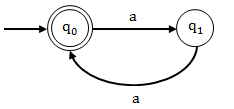For even no. of a’s finite automata can be constructed so it is regular.
Option (B):
L = { a2, a3, a5, a7, a11, a13, a17...................}
It is not regular language. A language is regular if the difference between two consecutive strings is same or we can say the language is in AP(arithmetic progression). But here if you see the difference between two consecutive strings/terms is not same. Hence it is not regular.
Statement (C):
L = {ab, aabb, ................... abab, baba, ababa, aababa, baabb, ..................}
It is also not regular language because the difference between two consecutive strings/terms is not same.
Statement (D): L = { a1, a4, a9, a16, a25, a36, a49...............}
It is also not regular language because the difference between two consecutive strings/terms is not same.
 Question 20
Consider the languages L1 = φ and L2 = {1}. Which one of the following represents L1* ∪ L1*L2* ?
 A {∈} B {∈, 1} C ϕ D 1*
Theory-of-Computation       Languages-and-Grammars
Question 20 Explanation:
As we know, for any regular expression R,
Rϕ = ϕR=ϕ
So L1 L2 * = ϕ
and L1 * = {ϕ}* = {ϵ}
So L1L2* ∪ L1* = {ϵ}
 Question 21
Given the following statements :
(A) A class of languages that is closed under union and complementation has to be closed under intersection.
(B) A class of languages that is closed under union and intersection has to be closed under complementation.
Which of the following options is correct?
 A Both (A) and (B) are false. B Both (A) and (B) are true. C (A) is true, (B) is false. D (A) is false, (B) is true.
Theory-of-Computation       Closure-Property
Question 21 Explanation:
be closed under intersection but if a language is not closing under either of union or complementation then it can or can not be closed under intersection.
So here, Statement (A) is true because regular language, context sensitive language and recursive language are closed under union and complementation and they are also closing under intersection but recursive enumerable language is closed under union but not under complementation but still it is closed under intersection.
Statement (B): The meaning of given statement is that if a class of languages that is closed under union and intersection then it has to be closed under complementation but if a language is not closing under either of union or intersection then it can or can not be closed under complementation.
So here, Statement (B) is false because regular language, context sensitive language, recursive language and recursively enumerable language are closed under union and intersection but only regular language, context sensitive language and recursive language are closed under complementation , recursively enumerable language is not closing under complementation after being closed under union and intersection.
Hence statement (B) is not true.
 Question 22
Let G = (V, T, S, P) be a context-free grammar such that every one of its productions is of the form A → v, with |v| = K > 1. The derivation tree for any W ∈ L(G) has a height h such that
 A logK|W| ≤ h ≤ logK((|W|-1) / k-1) B logK|W| ≤ h ≤ logK(K|W|) C logK|W| ≤ h ≤ K logK|W| D logK|W| ≤ h ≤ ((|W|-1) / k-1)
Theory-of-Computation       Context-Free-Grammar
Question 22 Explanation:
Min height of any tree is log_k |W|
For max height (since k>1, consider k=2) , hence it is CNF form and in CNF height is log_2 (|W|+1)
so in general it should be log_k ((|w|-1) / (k-1))
 Question 23
Given the following two languages : L1 = {an bn | n ≥ 0, n ≠ 100} L2 = {w ∈ {a, b, c}*| na(w) = nb(w) = nc(w)} Which of the following options is correct ?
 A Both L1 and L2 are not context free language B Both L1 and L2 are context free language. C L1 is context free language, L2 is not context free language. D L1 is not context free language, L2 is context free language.
Theory-of-Computation       Context-Free-Language
Question 23 Explanation:
Statement 1: L1 is a context free language because we can easily construct a pushdown automata which can push all a's onto the top of stack and then when b's comes as input for each "b" one "a" will be popped up. If number of a's is equal to number of b's then final stage is reached and string is accepted except the case when 100th "b" came as input we can go to a non final state and if after that any "b" came as input then the transaction can take place to a final state according to the equal number of b's and a's else we remain on that non-final state. So in this way a pushdown automata can be constructed for given language.
Hence L1 is a context free language.
Statement 2: L2 is not a context free language because we can push a's on the top of stack and when b's came as input we can pop one "a" for each "b" so in this way stack will get empty before comparing equal number of a, b and c. For comparing number of "c" the will be no "a or b" on the top of stack.
Hence L2 is not a context free language.
 Question 24
A recursive function h, is defined as follows :

h(m) = k, if m = 0

= 1, if m = 1

= 2 h(m – 1) + 4h(m – 2), if m ≥ 2

If the value of h(4) is 88 then the value of k is :

 A 0 B 1 C 2 D -1
Data-Structures       Recursion
Question 24 Explanation:
If k=2, 24 + 32 × 2 = 24 + 64 = 88Each iteration:
h(2) = 2h(1) + 4h(0) = 2 + 4k
h(3) = 2h(2) + 4h(1) = 4 + 8k + 4 = 8 + 8k
h(4) = 2h(3) + 4h(2) = 16 + 16k + 8 +16k = 32k + 24 = 88 (if k=2)
 Question 25
Suppose there are n stations in a slotted LAN. Each station attempts to transmit with a probability P in each time slot. The probability that only one station transmits in a given slot is _______.
 A nP(1 – P)n – 1 B nP C P(1 – P)n – 1 D nP(1 – P)n – 1
Engineering-Mathematics       Probability
Question 25 Explanation:
This question can be solved by binomial distribution, nC1P1(1-p)n-1 = nP(1-P)n-1
 Question 26
Station A uses 32 byte packets to transmit messages to station B using sliding window protocol. The round trip delay between A and B is 40 milliseconds and the bottleneck bandwidth on the path between A and B is 64 kbps. The optimal window size of A is ________.
 A 20 B 10 C 30 D 40
Computer-Networks       Sliding-Window-Protocol
Question 26 Explanation:
Given data,
L = 32 byte
Bandwidth = 64 kbps,
2Tp = 40 millisec
Tt = L / B = 32 *8 / 64*103 = 4 millisec
a= Tp/Tt
Optimal window size (N) = 1 + 2a
N = 1 + 2a ⇒ 1+ (40/4) ⇒ 11
The correct answer is 11. Option-B is more relevant.
 Question 27
Let G(x) be generator polynomial used for CRC checking. The condition that should be satisfied by G(x) to correct odd numbered error bits, will be:
 A (1 + x) is factor of G(x) B (1 – x) is factor of G(x) C (1 + x2) is factor of G(x) D x is factor of G(x)
Digital-Logic-Design       CRC
Question 27 Explanation:
Odd number of bit errors can be detected if G(x) contains (x+1) as a factor.
 Question 28
In a packet switching network, if the message size is 48 bytes and each packet contains a header of 3 bytes. If 24 packets are required to transmit the message, the packet size is ________.
 A 2 bytes B 1 bytes C 4 bytes D 5 bytes
Computer-Networks       Switching
Question 28 Explanation:
Given data,
-- Message size=48 bytes
-- 24 packets are carrying 48 bytes of data
-- Packet size=?
Step-1: One packet carries maximum size is= Message size / Transmission message size
= 48 / 24
= 2 bytes
Step-2: Packet size= One packet carries maximum size + new packet header size
= 2 + 3
= 5 byte
 Question 29
In RSA public key cryptosystem suppose n = p ∗ q where p and q are primes. (e, n) and (d, n) are public and private keys respectively. Let M be an integer such that o < M < n and φ(n) = (p – 1)(q – 1).

Which of the following equations represent RSA public key cryptosystem ?

I. C ≡ Me(mod n)

M ≡ (C)d(mod n)

II. ed ≡ 1(mod n)

III. ed ≡ 1(mod φ (n))

IV. C ≡ Me(mod φ (n))

M ≡ Cd(mod φ (n))

 A I and II B I and III C II and III D I and IV
Computer-Networks       RSA-algorithm
Question 29 Explanation:
To generate the encryption and decryption keys, we can proceed as follows.
1. Generate randomly two “large” primes p and q.
2. Compute n=pq and ∅=(p-1)(q-1).
3. Choose a number e so that
gcd(e,∅)=1
4. Find the multiplicative inverse of e modulo ∅, i.e., find d so that
ed≡1 (mod ∅)
This can be done efficiently using Euclid’s Extended Algorithm.
The encryption public key is KE=(n,e) and the decryption private key is KD=(n,d).
The encryption function is
E(M)=Me mod n
The decryption function is
D(M)=Md mod n
 Question 30
A node X on a 10 Mbps network is regulated by a token bucket. The token bucket is filled at a rate of 2 Mbps. Token bucket is initially filled with 16 megabits. The maximum duration taken by X to transmit at full rate of 10 Mbps is _________ secs.
 A 1 B 2 C 3 D 4
Computer-Networks       Congestion-Control
Question 30 Explanation:
Given data,
-- A node X on a 10 Mbps network is regulated by a token bucket.
-- Filled rate=2 Mbps
-- Initially token bucket filled time= 16 Megabits
-- Maximum duration time=?
Step-1: Maximum duration time= Initially token bucket filled time / (Regulated token time-Filled rate)
= 16 / (10-2)
= 16 / 8
= 2 seconds
 Question 31
The asymptotic upper bound solution of the recurrence T(n)= 2T(n/2)+(n/lg) is relation given by
 A O(n2) B O(n lg n) C O(n lg lg n) D O(lg lg n)
Algorithms       Recurrences
Question 31 Explanation:
→ The above recurrence is not in the form of Masters theorem. It doesn't apply since f(n)=n/logn is n polynomially smaller than nlog(base 2) 2-e for any epsilon>0.
We can apply after converting into n/logn as n*log-1n
2T(n/2)+n/logn is by taking a=2,b=2 ,k=1 ,p=-1
T(n) = Θ(nlog(base 2)2 log log n)
= O(n lg lg n)
 Question 32
Any decision tree that sorts n elements has height ________.
 A Ω(lg n) B Ω(n) C Ω(n lg n) D Ω(n2)
Algorithms       Sorting
Question 32 Explanation:
Theorem: Any decision tree that sorts n elements has height Ω(n lg n).
Proof:
→ The number of leaves l ≥ n!
→ Any binary tree of height h has ≤ 2h leaves.
→ Hence, n! ≤ l ≤ 2h
→ Take logs: h ≥ lg(n!)
→ Use Stirling’s approximation: n! > (n/e)n
→ We get:
h ≥ log(n/e)n
= nlg(n/e)
= nlg n - n lg e
= Ω(n lg n)
 Question 33
Red-black trees are one of many search tree schemes that are “balanced” in order to guarantee that basic dynamic-set operations take ________ time in the worst case.
 A O(1) B O(lg n) C O(n) D O(n lg n)
Algorithms       Algorithms Time-Complexity
 Question 34
The minimum number of scalar multiplication required, for parenthesization of a matrix-chain product whose sequence of dimensions for four matrices is <5, 10, 3, 12, 5> is
 A 630 B 580 C 480 D 405
Algorithms       Dynamic-Programming
Question 34 Explanation:Question 35
Dijkstra’s algorithm is based on
 A Divide and conquer paradigm B Dynamic programming C Greedy Approach D Backtracking paradigm
Algorithms       Greedy-approach
Question 35 Explanation:
Dijkstra’s algorithm is used on greedy approach for single source shortest path problem.
 Question 36
Match the following with respect to algorithm paradigms : 36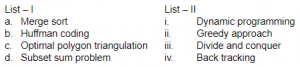A a-iii, b-i, c-ii, d-iv B a-ii, b-i, c-iv, d-iii C a-ii, b-i, c-iii, d-iv D b-iii, b-ii, c-i, d-iv
Algorithms       Sorting
Question 36 Explanation:
Merge sort→ Divide and conquer
Huffman coding→ Greedy approach
Optimal polygon triangulation→ Dynamic programming
Subset sum problem → Backtracking
Note: We can also use subset sum problem as well backtracking.
 Question 37
Abstraction and encapsulation are fundamental principles that underlie the object oriented approach to software development. What can you say about the following two statements ? I. Abstraction allows us to focus on what something does without considering the complexities of how it works. II. Encapsulation allows us to consider complex ideas while ignoring irrelevant detail that would confuse us.
 A Neither I nor II is correct. B Both I and II are correct. C Only II is correct. D Only I is correct.
Programming-in-c++       Properties
Question 37 Explanation:
Statement-I: FALSE
→ Abstraction is a process of hiding the implementation details and showing only functionality to the user.
→ The data type created by the data abstraction process is called abstract data type(ADT).
→ ADT is a class of objects whose logical behavior is defined by a set of values and a set of operations.
Statement-II: FALSE
→ Encapsulation is a mechanism to associate the code and data.
→ Encapsulation is wrapping up of data into single unit.
 Question 38
Given the array of integers ‘array’ shown below :What is the output of the following JAVA statements ?

int [ ] p = new int ;

int [ ] q = new int ;

for (int k = 0; k < 10; k ++)

p[k] = array [k];

q = p;

p = 20;

System.out.println (array  + “ : ” + q);

 A 20 : 20 B 18 : 18 C 18 : 20 D 20 : 18
OOPS       JAVA
Question 38 Explanation:Question 39
Consider the following JAVA program :
public class First {
public static int CBSE (int x) {
if (x < 100) x = CBSE (x + 10);
return (x – 1);
}
public static void main (String[] args){
System.out.print(First.CBSE(60));
}
}
What does this program print ?
 A 59 B 95 C 69 D 99
OOPS       JAVA
Question 39 Explanation:
Main program will call the function CBSE(60)
According to the function definition,
Function call CBSE(100) will give the value of 99,
100<100 condition is false and it will return x-1 which is 99
x=CBSE(90)
90<100 is true----> x=CBSE(100) and it will return x-1
CBSE(100) will return 99 to Function CBSE(90) and this function will return to 98(99-1).
Repeat this procedure,
CBSE(60) will return 95
 Question 40
Which of the following statement(s) with regard to an abstract class in JAVA is/are TRUE ?
I. An abstract class is one that is not used to create objects.
II. An abstract class is designed only to act as a base class to be inherited by other classes.
 A Only I B Only II C Neither I nor II D Both I and II
OOPS       JAVA
Question 40 Explanation:
Statement-I and II are TRUE
A class which is declared as abstract is known as an abstract class. It can have abstract and non-abstract methods. It needs to be extended and its method implemented. It cannot be instantiated.
Rules:
1. An abstract class must be declared with an abstract keyword.
2. It can have abstract and non-abstract methods.
3. It cannot be instantiated.
4. It can have constructors and static methods also.
5. It can have final methods which will force the subclass not to change the body of the method.
 Question 41
Which of the following HTML code will affect the vertical alignment of the table content?
 ABCDWeb-Technologies       HTML
Question 41 Explanation:Question 42
What can you say about the following statements ?
I. XML tags are case-insensitive.
II. In JavaScript, identifier names are case-sensitive.
III.Cascading Style Sheets (CSS) cannot be used with XML.
IV. All well-formed XML documents must contain a document type definition.
 A only I and II are false. B only III and IV are false. C only I and III are false. D All are incorrect.
Web-Technologies       XML
Question 42 Explanation:
FALSE: XML tags are case-sensitive.
FALSE: In JavaScript, identifier names are not case-sensitive.
FALSE: Cascading Style Sheets (CSS) can be used with XML.
FALSE: Somel well-formed XML documents must contain a document type definition.
 Question 43
Which of the following statement(s) is/are TRUE with regard to software testing ?
I. Regression testing technique ensures that the software product runs correctly after the changes during maintenance.
II. Equivalence partitioning is a white-box testing technique that divides the input domain of a program into classes of data from which test cases can be derived.
 A only I B only II C both I and II D neither I nor II
Software-Engineering       Software-testing
Question 43 Explanation:
Statement-1:
→ Regression testing is re-running functional and non-functional tests to ensure that previously developed and tested software still performs after a change. If not, that would be called a regression.
→ Changes that may require regression testing include bug fixes, software enhancements, configuration changes, and even substitution of electronic components.
→ As regression test suites tend to grow with each found defect, test automation is frequently involved.
Statement-2: Black box testing methods are
1. Graph-Based Testing Methods
2. Equivalence Partitioning: It is a black box testing technique that divides the input domain of a program into classes of data from which test cases can be derived.
3. Boundary Value Analysis
4. Orthogonal Array Testing
 Question 44
Which of the following are facts about a top-down software testing approach?
I. Top-down testing typically requires the tester to build method stubs.
II. Top-down testing typically requires the tester to build test drivers.
 A Only I B Only II C Both I and II D Neither I nor II
Software-Engineering       Software-testing
Question 44 Explanation:
Statement-1: TRUE: Top-down testing typically requires the tester to build method stubs.
Stub is a dummy subprogram. Stub uses the subordinate module’s interface, may do minimal data manipulation, provides verification of entry, and returns control to the module undergoing testing.
Statement-2: FALSE: Bottom up testing typically requires the tester to build test drivers.
Driver is nothing more than a “main program “ that accepts test case data, passes such data to the component(to be tested) and prints relevant results.
Driver is serve to replace modules that are subordinate to (called by) the component to be tested.
 Question 45
Match the terms related to Software Configuration Management (SCM) in List – I with the descriptions in List – II.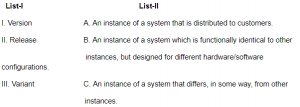A I-B, II-C, III-A B I-C, II-A, III-B C I-C, II-B, III-A D I-B, II-A, III-C
Software-Engineering       Software-configuration-management
Question 45 Explanation:
Version→ An instance of a system that differs, in some way, from other instances.
Release→ An instance of a system that is distributed to customers.
Variant→ An instance of a system which is functionally identical to other instances, but designed for different hardware/software configurations.
 Question 46
A software project was estimated at 352 Function Points (FP). A four person team will be assigned to this project consisting of an architect, two programmers, and a tester. The salary of the architect is 80,000 per month, the programmer ₹ 60,000 per month and the tester ₹ 50,000 per month. The average productivity for the team is 8 FP per person month. Which of the following represents the projected cost of the project ?
 A ₹ 28,16,000 B ₹ 20,90,000 C ₹ 26,95,000 D ₹ 27,50,000
Software-Engineering       Software-Development
Question 46 Explanation:
Given data,
-- Functional Point(FP)=352
-- Team=4 persons
-- Average each person(FP) productivity=8
-- Architect month salary=80,000
-- Programmers month salary=2*60,000
-- Tester month salary=50,000
-- projected cost of project=?
Step-1: Total number of months=Functional Point(FP) / (Team* Average each person(FP) productivity)
= 352 / (4*8)
= 352 / 32
= 11 months
Step-2: Total cost of the project= (one Architect month salary + 2*Programmers month salary + one tester month salary) * Total number of months
= (80000 + 2 * 60000 + 50000) * 11
= ₹ 27,50,000
 Question 47
Complete each of the following sentences in List – I on the left hand side by filling in the word or phrase from the List – II on the right hand side that best completes the sentence :A I-B, II-D, III-A, IV-C B I-B, II-D, III-C, IV-A C I-D, II-B, III-C, IV-A D I-D, II-B, III-A, IV-C
Software-Engineering       Software-testing
Question 47 Explanation:
→ Verification: Are we building the product right?
→ Validation: Are we building the right product?
→ Software debugging is the process of discovering the cause of a defect and fixing it.
→ is the process of demonstrating the existence.
 Question 48
A software company needs to develop a project that is estimated as 1000 function points and is planning to use JAVA as the programming language whose approximate lines of code per function point is accepted as 50. Considering a = 1.4 as multiplicative factor, b = 1.0 as exponential factor for the basic COCOMO effort equation and c = 3.0 as multiplicative factor, d = 0.33 as exponential factor for the basic COCOMO duration equation, approximately how long does the project take to complete ?
 A 11.2 months B 12.2 months C 13.2 months D 10.2 months
Software-Engineering       COCOMO-Model
Question 48 Explanation:
Given data,
-- lines of code(LOC)= 50,000 (or 50K)
-- Multiplicative value ‘a’=1.4
-- Exponential ‘b’=1.0
-- Basic COCOMO formula= a*(LOC in K)b
-- Multiplicative value ‘c’=3.0
-- Exponential ‘d’=0.33
-- Development=c*(basic COCOMO result)d
Step-1: Basic COCOMO formula= a*(LOC in K)b
= 1.4*(50)1.0
= 70 Step-2: Development= c*(basic COCOMO result)d
= 3(70)0.33
= 12.18 months
 Question 49
A memory management system has 64 pages with 512 bytes page size. Physical memory consists of 32 page frames. Number of bits required in logical and physical address are respectively:
 A 14 and 15 B 14 and 29 C 15 and 14 D 16 and 32
Operating-Systems       Memory-Management
Question 49 Explanation:
Given data,
-- Total number of pages=64
-- Page size=512
-- Page frames=32
Step-1: Logical address=Total number of pages*Page size
= 26*29
= 215
Step-2: Physical address= Page size*Page frames
= 29*25
= 214
 Question 50
Consider a disk queue with I/O requests on the following cylinders in their arriving order:

6, 10, 12, 54, 97, 73, 128, 15, 44, 110, 34, 45

The disk head is assumed to be at cylinder 23 and moving in the direction of decreasing number of cylinders. Total number of cylinders in the disk is 150. The disk head movement using SCAN-scheduling algorithm is:

 A 172 B 173 C 227 D 228
Operating-Systems       Disk-Scheduling
Question 50 Explanation: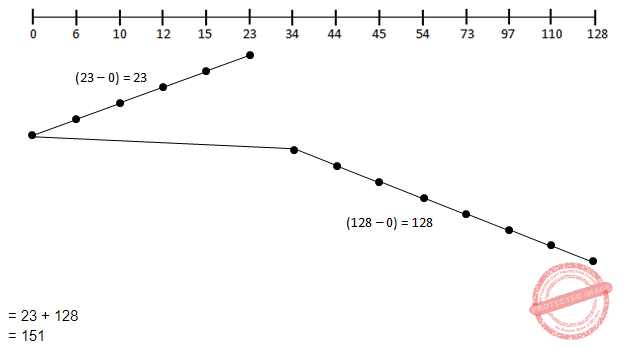Question 51
Match the following for Unix file system: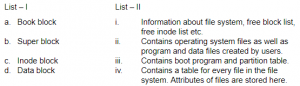A a-iii, b-i, c-ii, d-iv B a-iii, b-i, -iv, d-ii C a-iv, b-iii, c-ii, d-i D a-iv, b-iii, c-i, d-ii
Operating-Systems       File system
Question 51 Explanation:
Book block→ Contains boot program and partition table.
Super block→ Information about file system, free block list, free inode list etc.
Inode block→ Contains a table for every file in the file system. Attributes of files are stored here.
Data block→ Contains operating system files as well as program and data files created by users.
 Question 52
Some of the criteria for calculation of priority of a process are:
a. Processor utilization by an individual process.
b. Weight assigned to a user or group of users.
c. Processor utilization by a user or group of processes In fair share scheduler, priority is calculated based on:
 A only (a) and (b) B only (a) and (c) C (a), (b) and (c) D only (b) and (C)
Operating-Systems       Process-Scheduling
Question 52 Explanation:
Fair-share scheduling is a scheduling algorithm for computer operating systems in which the CPU usage is equally distributed among system users or groups, as opposed to equal distribution among processes.
 Question 53
 A If a user level thread of a process executes a system call, all threads in that process are blocked. B Scheduling is application dependent. C Thread switching doesn’t require kernel mode privileges. D The library procedures invoked for thread management in user level threads are local procedures.
Question 53 Explanation:
TRUE: If a user level thread of a process executes a system call, all threads in that process are blocked.
FALSE: Scheduling is application dependent.
FALSE: Thread switching doesn’t require kernel mode privileges.
FALSE: The library procedures invoked for thread management in user level threads are local procedures.
1. user-level threads package can be implemented on an Operating System that does not support threads.
2. User-level threads does not require modification to operating systems.
3. Simple Representation: Each thread is represented simply by a PC, registers, stack and a small control block, all stored in the user process address space.
4. Simple Management: This simply means that creating a thread, switching between threads and synchronization between threads can all be done without intervention of the kernel.
5. Fast and Efficient: Thread switching is not much more expensive than a procedure call.
1. User-Level threads are not a perfect solution as with everything else, they are a trade off.
2. There is a lack of coordination between threads and operating system kernel.
 Question 54
Which statement is not correct about “init” process in Unix?
 A It is generally the parent of the login shell. B It has PID 1. C It is the first process in the system. D Init forks and execs a ‘getty’ process at every port connected to a terminal.
Operating-Systems       System-Calls
Question 54 Explanation:
TRUE: It is generally the parent of the login shell.
TRUE: It has PID 1.
FALSE: It is the second process in the system.
TRUE: Init forks and execs a ‘getty’ process at every port connected to a terminal.
 Question 55

Consider following two rules R1 and R2 in logical reasoning in Artificial Intelligence (AI) :
R1 : From α ⊃ β
and α
------------------------
Inter β (is known as Modus Tollens (MT) )
-----------------------
R2 : From α ⊃ β
and ¬β
-------------------------
Inter ¬α ( is known as Modus Ponens (MP))
------------------------
 A Only R1 is correct. B Only R2 is correct. C Both R1 and R2 are correct. D Neither R1 nor R2 is correct.
Artificial-intelligence Linear-Distance        Logical-Reasoning
 Question 56
Consider the following AO graph:Which is the best node to expand next by AO* algorithm?
 A A B B C C D B and C
Artificial-intelligence       AO* -algorithm
Question 56 Explanation:f(n)=c(n)+h(n)
where
f(n)= Best path to reach the destination node
c(n)=Cost of path
h(n)= heuristic value of a node
Cost of choosing B or C is
=(22+3)+(24+2)
=51
Note: We add cost of B and C because they belongs to AND graph.
Cost of choosing A =42+4=46
Since cost of choosing node choosing B or C that’s why we will expand ‘A’.
 Question 57
In Artificial Intelligence (AI), what is present in the planning graph?
 A Sequence of levels B Literals C Variables D Heuristic estimates
Artificial-intelligence       Planning
Question 57 Explanation:
In Artificial Intelligence (AI), Sequence of levels is present in the planning graph.
→ A planning graph consists of a sequence of levels that correspond to time steps in the LEVELS plan, where level 0 is the initial state.
→ Each level contains a set of literals and a set of actions.
→ The literals are all those that could be true at that time step, depending on the actions executed at preceding time steps.
→ The actions are all those actions that could have their preconditions satisﬁed at that time step, depending on which of the literals actually hold.
→ The planning graph records only a restricted subset of the possible negative interactions among actions i.e.., it might be optimistic about the minimum number of time steps required for a literal to become true.
→ This number of steps in the planning graph provides a good estimate of how difﬁcult it is to achieve a given literal from the initial state.
→ The planning graph is deﬁned in such a way that it can be constructed very efﬁciently.
→ Planning graphs work only for propositional planning problems-ones with no variables.
 Question 58
What is the best method to go for the game playing problem?
 A Optimal Search B Random Search C Heuristic Search D Stratified Search
Artificial-intelligence       Heuristic-Search
Question 58 Explanation:
→ Heuristic search is the best method to go for the game playing problem
→ A heuristic is a method that might not always find the best solution but is guaranteed to find a good solution in reasonable time.
Example: Hill Climbing, Simulated Annealing, Best first search,A* algorithm,etc..,
 Question 59
Which of the following statements is true?
 A The sentence S is a logical consequence of S1,.., Sn if and only if S1 ∧ S2 ∧.... ∧ Sn → S is satisfiable. B The sentence S is a logical consequence of S1,..., Sn if and only if S1 ∧ S2 ∧........ ∧Sn → S is valid. C The sentence S is a logical consequence of S1,..., Sn if and only if S1 ∧ S2 ∧........ ∧ Sn ∧ S is consistent. D The sentence S is a logical consequence of S1,..., Sn if and only if S1 ∧ S2 ∧........ ∧ Sn ∧ S is inconsistent.
LPP       Satisfiability
 Question 60
The first order logic (FOL) statement ((R ∨ Q) ∧ (P ∨ ¬Q)) is equivalent to which of the following ?
 A ((R ∨ ¬Q) ∧ (P ∨ ¬Q) ∧ (R ∨ P)) B ((R ∨ Q) ∧ (P ∨ ¬Q) ∧ (R ∨ P)) C ((R ∨ Q) ∧ (P ∨ ¬Q) ∧ (R ∨ ¬P)) D ((R ∨ Q) ∧ (P ∨ ¬Q) ∧ ( ¬R ∨ P))
Engineering-Mathematics       Propositional-Logic
Question 60 Explanation:
Method-1: Boolean simplification
((R ∨ Q) ∧ (P ∨ Q’))
(R∨Q)∧(P∨Q’)∧(R∨P)
(PR ∨ Q’R ∨ PQ) ∧ (R∨P)
PR ∨ RQ’ ∨ PQR ∨ PR ∨ PRQ’ ∨ PQ
(RQ’∨PQ’R)∨(PQR∨PQ)∨PR
RQ’ ∨ PQ ∨ PR
((R ∨ Q) ∧ (P ∨ Q’) ∧ (R ∨ P))
Method-2: Using Truth table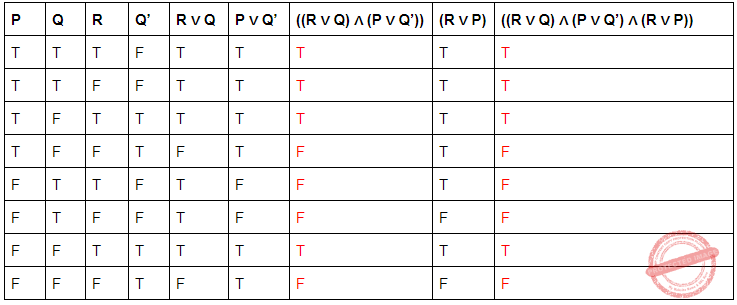Question 61
Given the following two statements :
A. L = {w|na(w) = nb(w)} is deterministic context free language, but not linear.
B. L = {an bn} ∪ {an b2n} is linear, but not deterministic context free language.
Which of the following options is correct ?
 A Both (A) and (B) are false. B Both (A) and (B) are true. C (A) is true, (B) is false. D (A) is false, (B) is true.
Theory-of-Computation       Context-Free-Language
Question 61 Explanation:
Statement-1:
A linear grammar is a context free grammar that has at most one nonterminal in the right hand side of each of its productions and language generated by linear grammar is known as a linear language.
ex-1: S→ aSb | ab
ex-2: S→ aS | Sb | ϵ
The grammar for (a)--> S→ϵ,S→SaSbS,S→SbSaS }

which is not linear. It is DCFL
Statement-2:
The example grammar is
S→ aSb | aSbb | ϵ
Hence it is linear language and it is not DCFL.
 Question 62
Which of the following pairs have different expressive power?
 A Single-tape-turing machine and multi-dimensional turing machine. B Multi-tape turing machine and multi-dimensional turing machine. C Deterministic pushdown automata and non-deterministic pushdown automata. D Deterministic finite automata and Non-deterministic finite automata
Theory-of-Computation       Turing-machines
Question 62 Explanation:
Option(A): It is incorrect option because using multi-dimensional turing machine we can speed up the process of accepting the language but it does not mean that Single-tape-turing machine can't accept the string that multidimensional turing machine accepts.

Option(B): is also incorrect because both machines are same so they can accept the same string with same speed.

Option(C) : It is correct option because Non deterministic pushdown automata is more powerful than deterministic pushdown automata because by using non-deterministic pushdown automata L= {ww | w belongs to (a,b)*} can be accepted but the same language can't be accepted using deterministic pushdown automata.

Option(D) : it is incorrect option because DFA and NDFA both are equivalent to each other i.e. we can convert DFA into NFA and NFA into DFA. DFA and NFA are equal in powers and can accept the same strings.

 Question 63
Which of the following statements is false?
 A Every context-sensitive language is recursive. B The set of all languages that are not recursively enumerable is countable. C The family of recursively enumerable languages is closed under union. D The families of recursively enumerable and recursive languages are closed under reversal.
Theory-of-Computation       Languages-and-Grammars
Question 63 Explanation:
Option(A) : it is correct option because L= {an bn cn} is a context sensitive language and it can also be accepted by the turing machine. Hence every CSL also recursive language.

Option(B): It is incorrect option.

Option(C): Yes it is true that recursively enumerable languages are closed under union.

Option(D): It is a correct option because recursively enumerable and recursive languages are closed under reversal.

NOTE: The difference between recursive and recursively enumerable language is that if a language is recursive then there will be definitely a turing machine which will halt and will say whether the string is accepted or not. But if a language is recursively enumerable then the turing machine will halt if the string is accepted but it is not necessary that the turing machine will halt to indicate that the string is not accepted. in this case the user might end up in infinite wait by thinking that turing machine will halt string acceptance process is still in progress.

 Question 64
Let C be a binary linear code with minimum distance 2t + 1 then it can correct upto _____ bits of error.
 A t + 1 B t C t - 2 D t / 2
Digital-Logic-Design       Number-Systems
Question 64 Explanation:
A binary linear code with minimum distance 2t + 1 then it can correct up to ‘T’ bits of error.
 Question 65
A t-error correcting q-nary linear code must satisfy:Where M is the number of code words and X is
 A qn B qt C q-n D q-t
LPP       Linear-Code
 Question 66
Names of some of the Operating Systems are given below:
(a) MS-DOS
(b) XENIX
(c) OS/2
In the above list, following operating systems didn’t provide multi user facility.
 A (a) only B (a) and (b) only C (b) and (c) only D (a), (b) and (c)
Operating-Systems       Basics
Question 66 Explanation:
MS-DOS→ Single user operating system
XENIX→ Multi user operating system. XENIX is a multi-user solution that allows multiple users to be attached via inexpensive terminals to a single machine, thereby allowing the users to share the resources of the machine.
OS/2→ Multi user operating system.
 Question 67
From the given data below :
a b b a a b b a a b
which one of the following is not a word in the dictionary created by LZ-coding (the initial words are a, b)?
 A a b B b b C b a D b a a b E None of the above
Image-Processing       LZ-Coding
Question 67 Explanation:
Lempel–Ziv–Welch is a universal lossless data compression algorithm created by Abraham Lempel, Jacob Ziv, and Terry Welch
abbaabbaab
⇒ Dictionary created by L2 - coding will contain words:
a | b | ba | ab | baa | b
b - already present in dictionary
So the words are a, b, ba, ab, baa.
⇒ Hence ‘B’ and ‘D’ both are not present in the dictionary.
Note: (B) and (D) is correct answers
 Question 68
With respect to a loop in the transportation table, which one of the following is not correct?
 A Every loop has an odd no. of cells and at least 5. B Closed loops may or may not be square in shape. C All the cells in the loop that have a plus or minus sign, except the starting cell, must be occupied cells. D Every loop has an even no. of cells and at least four.
LPP       Transportation-Problem
 Question 69
At which of the following stage(s), the degeneracy do not occur in transportation problem ? (m, n represents number of sources and destinations respectively)
(a) While the values of dual variables ui and vj cannot be computed.
(b) While obtaining an initial solution, we may have less than m + n – 1 allocations.
(c) At any stage while moving towards optimal solution, when two or more occupied cells with the same minimum allocation become unoccupied simultaneously.
(d) At a stage when the no. of +ve allocation is exactly m + n – 1.
 A (a), (b) and (c) B (a), (c) and (d) C (a) and (d) D (a), (b), (c) and (d)
LPP       Transportation-Problem
 Question 70
Consider the following LPP :
Min. Z = X1 + X2 + X3
Subject to 3X1 + 4X3 ≤ 5
5X1 + X2 + 6X3 = 7
8X1 + 9X3 ≥ 2,
X1, X2, X3 ≥ 0
The standard form of this LPP shall be :
 ABCDLPP       Standard-Form
Question 70 Explanation: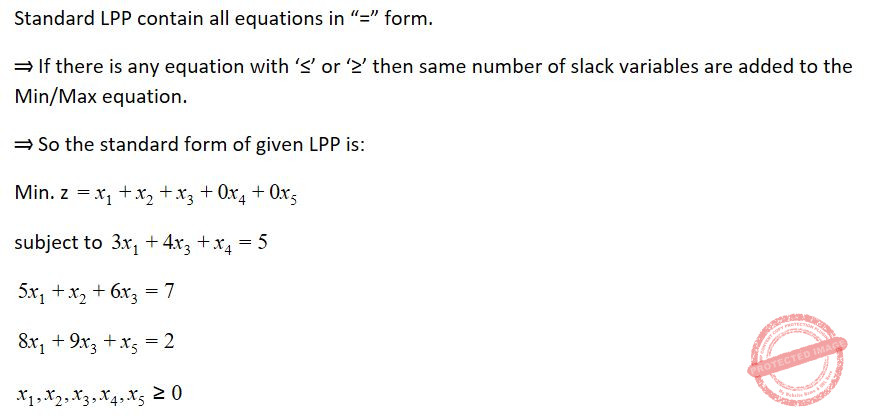Question 71
Let R and S be two fuzzy relations defined as :Then, the resulting relation, T, which relates elements of universe x to the elements of universe z using max-min composition is given by :
 A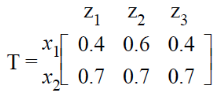B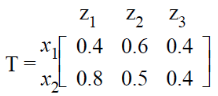CDArtificial-intelligence       Fuzzy-sets
Question 71 Explanation:Question 72
A neuron with 3 inputs has the weight vector [0.2 –0.1 0.1]T and a bias θ = 0. If the input vector is X = [0.2 0.4 0.2]T then the total input to the neuron is :
 A 0.20 B 1.0 C 0.02 D –1.0
Artificial-intelligence       Neural-Networks
Question 72 Explanation:
Total input to neuron f(x)=(WTXX)+Ⲑ
=(0.2*0.2)+(-0.1*0.4)+(0.1*0.2)
=0.04-0.04+0.02
=0.02
 Question 73
Which of the following neural networks uses supervised learning ?
(A) Multilayer perceptron
(B) Self organizing feature map
(C) Hopfield network

 A (A) only B (B) only C (A) and (B) only D (A) and (C) only
Artificial-intelligence       Neural-Networks
Question 73 Explanation:Question 74
Unix command to change the case of first three lines of file “shortlist” from lower to upper
 A \$ tr ‘[a – z]’ ‘[A – Z]’ shortlist ¦ head-3 B \$ head -3 shortlist ¦ tr ‘[a – z]’ ‘[A – Z]’ C \$ tr head -3 shortlist ‘[A – Z]’ ‘[a – z]’ D \$ tr shortlist head -3 ‘[a – z]’ ‘[A – Z]’
Operating-Systems       UNIX-Operating-System
Question 74 Explanation:
Head: The head command is a command-line utility for outputting the first part of files given to it via standard input. It writes results to standard output. By default head returns the first ten lines of each file that it is given.
tr: Translates lowercase to uppercase (or) uppercase to lowercase.
Option-B will display first three lines of file “shortlist” from lower to upper
 Question 75
Match the following vi commands in Unix:A a-ii, b-iii, c-i, d-iv B a-iv, b-iii, c-ii, d-i C a-iii, b-iv, c-i, d-ii D a-iii, b-i, c-iv, d-ii
Operating-Systems       UNIX-Operating-System
Question 75 Explanation:
: w → saves file and remains in editing mode
: x → saves the file and quits editingmode
: q → quits editing mode and no changes are saved to the file
: sh→ escapes unix shell
There are 75 questions to complete.